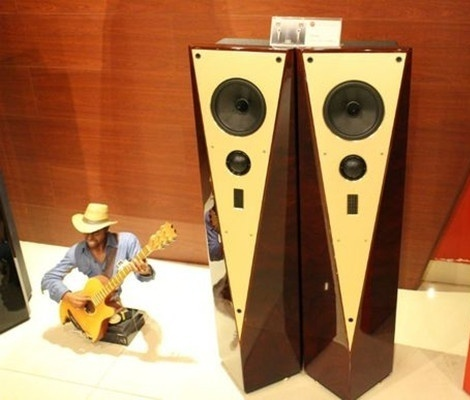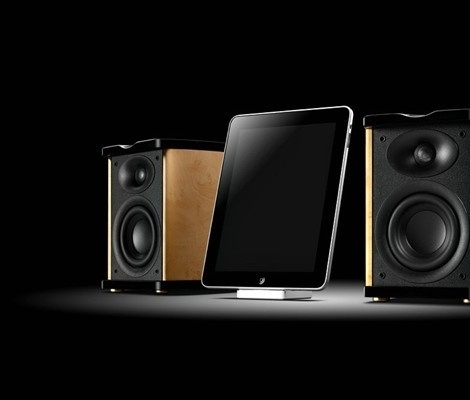|

# 惠威t200a深度测评介绍及报价

一说到音箱品牌，相信大家一定会想到惠威这个音箱品牌，没错，今天小编要为大家介绍的就是惠威t200a音箱，和这个型号一起上市的还有T200，这款音箱呢是无源箱，使用的时候需要搭配功放来进行，今天小编在这里就不给大家详细介绍了，下面着重介绍的是惠威t200a这款音箱产品的详细评测以及市场上的最新报价情况，想了解的朋友就跟着小编往下看吧。惠威t200a详细评测介绍

外观设计

首先它在包装上就非常讲究，没有采用塑料包装方式，而是用的防尘布袋来包装，这种布袋包装其实更加方便实用，能反复多次使用，而且在不用时也可以罩起音箱来防尘。T200A的两个主箱体完全相同，造型突破传统异常前卫，主色调为黑白二色，对比异常鲜明，颇具视觉冲击力。外形上是突破了传统的立方体形状，前面板是由三个三角形构成的多面体形，颇具时尚家居电器的味道，因此更容易在家庭和个人用户的市场上打开突破口。

整个三个面的前板都是黑色钢琴漆，正中的前板上是一个单独的白色版由螺丝固定上的，可以拆下来，其上留有中音和高音扬声器的圆孔，而且下方还带有一个LED指示灯的圆孔，在音箱工作时，黑色的面罩下隐约中泛着蓝色的亮光，为死气沉沉的音箱增加了几分生气。

扬声器

M5N采用大功率耐高温无涡流损耗Kapton音圈骨架和耐高温SV线音圈，屏蔽式磁路系统和长冲程线性位移设计，惠威特有对称磁场(SMD)驱动技术使音圈处于一个对称的驱动磁场之中，从而获得对称均衡的驱动力，能明显减少音圈的电感以降低反电动势对输出级的对峙，扬声器的控制特性因此得以改善，音色更加丰满有力，失真度也随之降低。

M5N单元适用于小型倒相式两分频书架箱的中低频，家庭影院主音箱、中置、环绕及超低频音箱，同样可用在电脑多媒体音箱中，M5N单元频响曲线如图，从80Hz到1KHz频响曲线相当平滑，以86dB为平均值，高未超过4dB，低未过2dB，以平均值下降6dB声强降低一半来看，即使在50Hz时，M5N也具有不错的表现力。其实国内的金属膜扬声器的制造水平在国际上并不突出，大家也不必把这个M5N中音单元想象的过分完美。功能设计

在功能设计方面，T200A除了开关以外，只提供了总音量这唯一的调节，但是这对于书架式监听型音响来说也未尝不是一件好事情，因为2.0监听箱的摆放位置相对固定，不同用户对它的定制差异也很小，这样设计省去了普通用户还需要自己调谐高低音所带来的不必要麻烦。

结构设计

T200A放弃了传统多媒体音箱都采用主副箱的设计思想，而采用了单声道独立功放的设计，因此在从外观、箱体结构到内部电路设计，左右两个箱体都是完全一样的。这样做可以保证两个箱体声音特性的完全一致，是一种绝对意义上的进步，但是在平时使用中的调节上无疑要麻烦一些。

箱体为倒相式设计，倒相口开口向后，位于后板散热片下方。箱体以倒立稍向后倾的三角形做为正面声障板，障板辐射面积小，箱体内部空间大，在设计上保证了低频部分的演绎效果。T200A外形的不规整使得内部空间也并不规则起来，内部结构前后不对称，但是依旧保证了左右的对称，因此可以避免长方体箱体内因各个面声音反射的同步对称而造成箱体内产生驻波的可能，从而也维持了输出声音的真实性。惠威这种把箱体设计成三角形非对称箱体，改变了箱体各反射面的反射轨迹，分散反射声能量，从而使得叠加聚焦音染能降到最低限度。

T200A的箱体异常沉重，感觉上要比同样大的多媒体音箱重量高出一倍，箱体板材为约15mm厚的高密度板材，而并非普通多媒体音箱所有的复合密度板，从箱体制作上避免了声波给箱体造成谐振的可能。惠威t200a最新报价

这款音箱上市的时间还是比较早的，停产与2008年6月，但是市场上仍有销售，目前这款惠威t200a音箱产品的最新报价为2250元。(价格来源于网络，仅供参考)总的来说这款惠威t200a面向的高端用户的一款产品，当然也属于入门级的监听音箱，结合市场上的最新报价来看性价比还是挺高的，非常值得大家入手哦，至于惠威t200a这款产品到底值不值得市场上的这个价格，小编在这里也不多评价，只要适合自己就是最好的。如果你对音箱的外形设计以及音箱系统上有着较高的要求，那么相信惠威t200a这款音箱会是你最好的选择。

`声明：本文由入驻焦点开放平台的作者撰写，除焦点官方账号外，观点仅代表作者本人，不代表焦点立场错误信息举报电话： 400-099-0099，邮箱：jubao@vip.sohu.com，或点此进行意见反馈，或点此进行举报投诉。`A B C D E F G H J K L M N P Q R S T W X Y Z
A - B - C - D - E
• A
• 鞍山
• 安庆
• 安阳
• 安顺
• 安康
• 澳门
• B
• 北京
• 保定
• 包头
• 巴彦淖尔
• 本溪
• 蚌埠
• 亳州
• 滨州
• 北海
• 百色
• 巴中
• 毕节
• 保山
• 宝鸡
• 白银
• 巴州
• C
• 承德
• 沧州
• 长治
• 赤峰
• 朝阳
• 长春
• 常州
• 滁州
• 池州
• 长沙
• 常德
• 郴州
• 潮州
• 崇左
• 重庆
• 成都
• 楚雄
• 昌都
• 慈溪
• 常熟
• D
• 大同
• 大连
• 丹东
• 大庆
• 东营
• 德州
• 东莞
• 德阳
• 达州
• 大理
• 德宏
• 定西
• 儋州
• 东平
• E
• 鄂尔多斯
• 鄂州
• 恩施
F - G - H - I - J
• F
• 抚顺
• 阜新
• 阜阳
• 福州
• 抚州
• 佛山
• 防城港
• G
• 赣州
• 广州
• 桂林
• 贵港
• 广元
• 广安
• 贵阳
• 固原
• H
• 邯郸
• 衡水
• 呼和浩特
• 呼伦贝尔
• 葫芦岛
• 哈尔滨
• 黑河
• 淮安
• 杭州
• 湖州
• 合肥
• 淮南
• 淮北
• 黄山
• 菏泽
• 鹤壁
• 黄石
• 黄冈
• 衡阳
• 怀化
• 惠州
• 河源
• 贺州
• 河池
• 海口
• 红河
• 汉中
• 海东
• I
• J
• 晋中
• 锦州
• 吉林
• 鸡西
• 佳木斯
• 嘉兴
• 金华
• 景德镇
• 九江
• 吉安
• 济南
• 济宁
• 焦作
• 荆门
• 荆州
• 江门
• 揭阳
• 金昌
• 酒泉
• 嘉峪关
K - L - M - N - P
• K
• 开封
• 昆明
• 昆山
• L
• 廊坊
• 临汾
• 辽阳
• 连云港
• 丽水
• 六安
• 龙岩
• 莱芜
• 临沂
• 聊城
• 洛阳
• 漯河
• 娄底
• 柳州
• 来宾
• 泸州
• 乐山
• 六盘水
• 丽江
• 临沧
• 拉萨
• 林芝
• 兰州
• 陇南
• M
• 牡丹江
• 马鞍山
• 茂名
• 梅州
• 绵阳
• 眉山
• N
• 南京
• 南通
• 宁波
• 南平
• 宁德
• 南昌
• 南阳
• 南宁
• 内江
• 南充
• P
• 盘锦
• 莆田
• 平顶山
• 濮阳
• 攀枝花
• 普洱
• 平凉
Q - R - S - T - W
• Q
• 秦皇岛
• 齐齐哈尔
• 衢州
• 泉州
• 青岛
• 清远
• 钦州
• 黔南
• 曲靖
• 庆阳
• R
• 日照
• 日喀则
• S
• 石家庄
• 沈阳
• 双鸭山
• 绥化
• 上海
• 苏州
• 宿迁
• 绍兴
• 宿州
• 三明
• 上饶
• 三门峡
• 商丘
• 十堰
• 随州
• 邵阳
• 韶关
• 深圳
• 汕头
• 汕尾
• 三亚
• 三沙
• 遂宁
• 山南
• 商洛
• 石嘴山
• T
• 天津
• 唐山
• 太原
• 通辽
• 铁岭
• 泰州
• 台州
• 铜陵
• 泰安
• 铜仁
• 铜川
• 天水
• 天门
• W
• 乌海
• 乌兰察布
• 无锡
• 温州
• 芜湖
• 潍坊
• 威海
• 武汉
• 梧州
• 渭南
• 武威
• 吴忠
• 乌鲁木齐
X - Y - Z
• X
• 邢台
• 徐州
• 宣城
• 厦门
• 新乡
• 许昌
• 信阳
• 襄阳
• 孝感
• 咸宁
• 湘潭
• 湘西
• 西双版纳
• 西安
• 咸阳
• 西宁
• 仙桃
• 西昌
• Y
• 运城
• 营口
• 盐城
• 扬州
• 鹰潭
• 宜春
• 烟台
• 宜昌
• 岳阳
• 益阳
• 永州
• 阳江
• 云浮
• 玉林
• 宜宾
• 雅安
• 玉溪
• 延安
• 榆林
• 银川
• Z
• 张家口
• 镇江
• 舟山
• 漳州
• 淄博
• 枣庄
• 郑州
• 周口
• 驻马店
• 株洲
• 张家界
• 珠海
• 湛江
• 肇庆
• 中山
• 自贡
• 资阳
• 遵义
• 昭通
• 张掖
• 中卫

1室1厅1厨1卫1阳台

1
2
3
4
5

0
1
2

1

1

0
1
2
3报名成功，资料已提交审核A B C D E F G H J K L M N P Q R S T W X Y Z
A - B - C - D - E
• A
• 鞍山
• 安庆
• 安阳
• 安顺
• 安康
• 澳门
• B
• 北京
• 保定
• 包头
• 巴彦淖尔
• 本溪
• 蚌埠
• 亳州
• 滨州
• 北海
• 百色
• 巴中
• 毕节
• 保山
• 宝鸡
• 白银
• 巴州
• C
• 承德
• 沧州
• 长治
• 赤峰
• 朝阳
• 长春
• 常州
• 滁州
• 池州
• 长沙
• 常德
• 郴州
• 潮州
• 崇左
• 重庆
• 成都
• 楚雄
• 昌都
• 慈溪
• 常熟
• D
• 大同
• 大连
• 丹东
• 大庆
• 东营
• 德州
• 东莞
• 德阳
• 达州
• 大理
• 德宏
• 定西
• 儋州
• 东平
• E
• 鄂尔多斯
• 鄂州
• 恩施
F - G - H - I - J
• F
• 抚顺
• 阜新
• 阜阳
• 福州
• 抚州
• 佛山
• 防城港
• G
• 赣州
• 广州
• 桂林
• 贵港
• 广元
• 广安
• 贵阳
• 固原
• H
• 邯郸
• 衡水
• 呼和浩特
• 呼伦贝尔
• 葫芦岛
• 哈尔滨
• 黑河
• 淮安
• 杭州
• 湖州
• 合肥
• 淮南
• 淮北
• 黄山
• 菏泽
• 鹤壁
• 黄石
• 黄冈
• 衡阳
• 怀化
• 惠州
• 河源
• 贺州
• 河池
• 海口
• 红河
• 汉中
• 海东
• I
• J
• 晋中
• 锦州
• 吉林
• 鸡西
• 佳木斯
• 嘉兴
• 金华
• 景德镇
• 九江
• 吉安
• 济南
• 济宁
• 焦作
• 荆门
• 荆州
• 江门
• 揭阳
• 金昌
• 酒泉
• 嘉峪关
K - L - M - N - P
• K
• 开封
• 昆明
• 昆山
• L
• 廊坊
• 临汾
• 辽阳
• 连云港
• 丽水
• 六安
• 龙岩
• 莱芜
• 临沂
• 聊城
• 洛阳
• 漯河
• 娄底
• 柳州
• 来宾
• 泸州
• 乐山
• 六盘水
• 丽江
• 临沧
• 拉萨
• 林芝
• 兰州
• 陇南
• M
• 牡丹江
• 马鞍山
• 茂名
• 梅州
• 绵阳
• 眉山
• N
• 南京
• 南通
• 宁波
• 南平
• 宁德
• 南昌
• 南阳
• 南宁
• 内江
• 南充
• P
• 盘锦
• 莆田
• 平顶山
• 濮阳
• 攀枝花
• 普洱
• 平凉
Q - R - S - T - W
• Q
• 秦皇岛
• 齐齐哈尔
• 衢州
• 泉州
• 青岛
• 清远
• 钦州
• 黔南
• 曲靖
• 庆阳
• R
• 日照
• 日喀则
• S
• 石家庄
• 沈阳
• 双鸭山
• 绥化
• 上海
• 苏州
• 宿迁
• 绍兴
• 宿州
• 三明
• 上饶
• 三门峡
• 商丘
• 十堰
• 随州
• 邵阳
• 韶关
• 深圳
• 汕头
• 汕尾
• 三亚
• 三沙
• 遂宁
• 山南
• 商洛
• 石嘴山
• T
• 天津
• 唐山
• 太原
• 通辽
• 铁岭
• 泰州
• 台州
• 铜陵
• 泰安
• 铜仁
• 铜川
• 天水
• 天门
• W
• 乌海
• 乌兰察布
• 无锡
• 温州
• 芜湖
• 潍坊
• 威海
• 武汉
• 梧州
• 渭南
• 武威
• 吴忠
• 乌鲁木齐
X - Y - Z
• X
• 邢台
• 徐州
• 宣城
• 厦门
• 新乡
• 许昌
• 信阳
• 襄阳
• 孝感
• 咸宁
• 湘潭
• 湘西
• 西双版纳
• 西安
• 咸阳
• 西宁
• 仙桃
• 西昌
• Y
• 运城
• 营口
• 盐城
• 扬州
• 鹰潭
• 宜春
• 烟台
• 宜昌
• 岳阳
• 益阳
• 永州
• 阳江
• 云浮
• 玉林
• 宜宾
• 雅安
• 玉溪
• 延安
• 榆林
• 银川
• Z
• 张家口
• 镇江
• 舟山
• 漳州
• 淄博
• 枣庄
• 郑州
• 周口
• 驻马店
• 株洲
• 张家界
• 珠海
• 湛江
• 肇庆
• 中山
• 自贡
• 资阳
• 遵义
• 昭通
• 张掖
• 中卫• 手机• 分享
• 设计
免费设计
• 计算器
装修计算器
• 入驻
合作入驻
• 联系
联系我们
• 置顶
返回顶部